# Solution for submission 152146

A detailed solution for submission 152146 submitted for challenge Starship Detection

## Starter Code for Starship Detection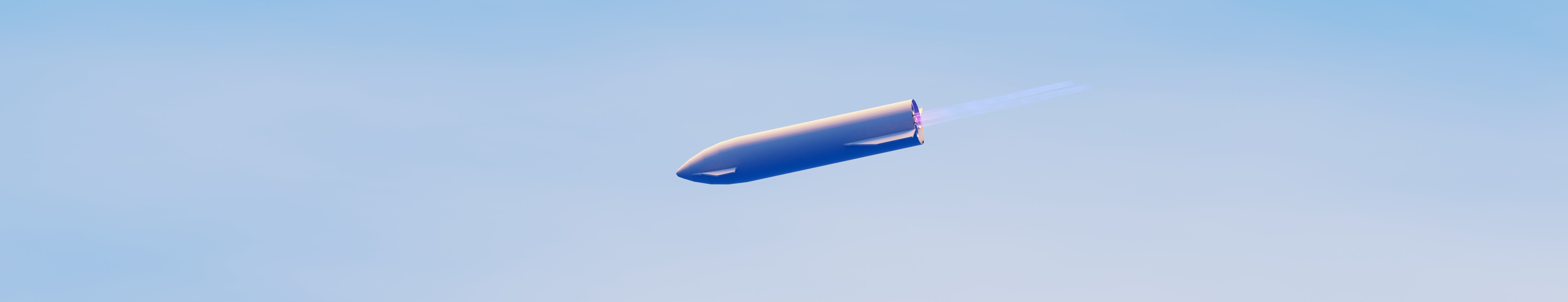### What we are going to Learn¶

• Basic Image Preprocessing with OpenCV
• Fixing Contours on an image and getting bounding boxes.
• Testing and Submitting the Results to the Challenge.

Note : Create a copy of the notebook and use the copy for submission. Go to File > Save a Copy in Drive to create a new copy

# Setting up Environment¶

So we will first need to download the python library by AIcrowd that will allow us to download the dataset by just inputting the API key.

In :
```!pip install aicrowd-cli

```
```Collecting aicrowd-cli
|████████████████████████████████| 43 kB 904 kB/s
Collecting requests<3,>=2.25.1
|████████████████████████████████| 62 kB 653 kB/s
Collecting tqdm<5,>=4.56.0
|████████████████████████████████| 76 kB 3.1 MB/s
Collecting GitPython==3.1.18
|████████████████████████████████| 170 kB 8.8 MB/s
Collecting rich<11,>=10.0.0
|████████████████████████████████| 208 kB 9.2 MB/s
Requirement already satisfied: click<8,>=7.1.2 in /usr/local/lib/python3.7/dist-packages (from aicrowd-cli) (7.1.2)
Collecting requests-toolbelt<1,>=0.9.1
|████████████████████████████████| 54 kB 2.1 MB/s
Requirement already satisfied: toml<1,>=0.10.2 in /usr/local/lib/python3.7/dist-packages (from aicrowd-cli) (0.10.2)
Requirement already satisfied: typing-extensions>=3.7.4.0 in /usr/local/lib/python3.7/dist-packages (from GitPython==3.1.18->aicrowd-cli) (3.7.4.3)
Collecting gitdb<5,>=4.0.1
|████████████████████████████████| 63 kB 1.4 MB/s
Collecting smmap<5,>=3.0.1
Requirement already satisfied: urllib3<1.27,>=1.21.1 in /usr/local/lib/python3.7/dist-packages (from requests<3,>=2.25.1->aicrowd-cli) (1.24.3)
Requirement already satisfied: charset-normalizer~=2.0.0 in /usr/local/lib/python3.7/dist-packages (from requests<3,>=2.25.1->aicrowd-cli) (2.0.2)
Requirement already satisfied: idna<4,>=2.5 in /usr/local/lib/python3.7/dist-packages (from requests<3,>=2.25.1->aicrowd-cli) (2.10)
Requirement already satisfied: certifi>=2017.4.17 in /usr/local/lib/python3.7/dist-packages (from requests<3,>=2.25.1->aicrowd-cli) (2021.5.30)
Collecting colorama<0.5.0,>=0.4.0
Collecting commonmark<0.10.0,>=0.9.0
|████████████████████████████████| 51 kB 5.1 MB/s
Requirement already satisfied: pygments<3.0.0,>=2.6.0 in /usr/local/lib/python3.7/dist-packages (from rich<11,>=10.0.0->aicrowd-cli) (2.6.1)
Installing collected packages: smmap, requests, gitdb, commonmark, colorama, tqdm, rich, requests-toolbelt, GitPython, aicrowd-cli
Attempting uninstall: requests
Found existing installation: requests 2.23.0
Uninstalling requests-2.23.0:
Successfully uninstalled requests-2.23.0
Attempting uninstall: tqdm
Found existing installation: tqdm 4.41.1
Uninstalling tqdm-4.41.1:
Successfully uninstalled tqdm-4.41.1
ERROR: pip's dependency resolver does not currently take into account all the packages that are installed. This behaviour is the source of the following dependency conflicts.
google-colab 1.0.0 requires requests~=2.23.0, but you have requests 2.26.0 which is incompatible.
datascience 0.10.6 requires folium==0.2.1, but you have folium 0.8.3 which is incompatible.
Successfully installed GitPython-3.1.18 aicrowd-cli-0.1.9 colorama-0.4.4 commonmark-0.9.1 gitdb-4.0.7 requests-2.26.0 requests-toolbelt-0.9.1 rich-10.6.0 smmap-4.0.0 tqdm-4.62.0
```
In :
```%aicrowd login --api-key c18a6c4b759fa2df29481af274df6942
```
```API Key valid
Saved API Key successfully!
```
In :
```# Downloading the Dataset
!rm -rf data
!mkdir data
%aicrowd ds dl -c starship-detection -o data
```
In :
```!unzip data/data.zip -d /content/images >> /dev/null
```
In :
```!pip uninstall scikit-image -y
!pip install scikit-image
```
```Found existing installation: scikit-image 0.16.2
Uninstalling scikit-image-0.16.2:
Successfully uninstalled scikit-image-0.16.2
Collecting scikit-image
|████████████████████████████████| 29.2 MB 2.5 MB/s
Requirement already satisfied: imageio>=2.3.0 in /usr/local/lib/python3.7/dist-packages (from scikit-image) (2.4.1)
Requirement already satisfied: scipy>=1.0.1 in /usr/local/lib/python3.7/dist-packages (from scikit-image) (1.4.1)
Requirement already satisfied: PyWavelets>=1.1.1 in /usr/local/lib/python3.7/dist-packages (from scikit-image) (1.1.1)
Requirement already satisfied: matplotlib!=3.0.0,>=2.0.0 in /usr/local/lib/python3.7/dist-packages (from scikit-image) (3.2.2)
Requirement already satisfied: numpy>=1.16.5 in /usr/local/lib/python3.7/dist-packages (from scikit-image) (1.19.5)
Requirement already satisfied: tifffile>=2019.7.26 in /usr/local/lib/python3.7/dist-packages (from scikit-image) (2021.7.2)
Requirement already satisfied: pillow!=7.1.0,!=7.1.1,>=4.3.0 in /usr/local/lib/python3.7/dist-packages (from scikit-image) (7.1.2)
Requirement already satisfied: networkx>=2.0 in /usr/local/lib/python3.7/dist-packages (from scikit-image) (2.5.1)
Requirement already satisfied: pyparsing!=2.0.4,!=2.1.2,!=2.1.6,>=2.0.1 in /usr/local/lib/python3.7/dist-packages (from matplotlib!=3.0.0,>=2.0.0->scikit-image) (2.4.7)
Requirement already satisfied: python-dateutil>=2.1 in /usr/local/lib/python3.7/dist-packages (from matplotlib!=3.0.0,>=2.0.0->scikit-image) (2.8.1)
Requirement already satisfied: cycler>=0.10 in /usr/local/lib/python3.7/dist-packages (from matplotlib!=3.0.0,>=2.0.0->scikit-image) (0.10.0)
Requirement already satisfied: kiwisolver>=1.0.1 in /usr/local/lib/python3.7/dist-packages (from matplotlib!=3.0.0,>=2.0.0->scikit-image) (1.3.1)
Requirement already satisfied: six in /usr/local/lib/python3.7/dist-packages (from cycler>=0.10->matplotlib!=3.0.0,>=2.0.0->scikit-image) (1.15.0)
Requirement already satisfied: decorator<5,>=4.3 in /usr/local/lib/python3.7/dist-packages (from networkx>=2.0->scikit-image) (4.4.2)
Installing collected packages: scikit-image
ERROR: pip's dependency resolver does not currently take into account all the packages that are installed. This behaviour is the source of the following dependency conflicts.
albumentations 0.1.12 requires imgaug<0.2.7,>=0.2.5, but you have imgaug 0.2.9 which is incompatible.
Successfully installed scikit-image-0.18.2
```

# Importing Libraries¶

In :
```# Image Reading & Preprocessing
import skimage.io
import matplotlib.pyplot as plt
import numpy as np

# Misc.
import pandas as pd
from tqdm.notebook import tqdm
import os
from natsort import natsorted
from skimage.color import rgb2gray
from skimage.filters import sobel
from skimage.draw import rectangle
from skimage.measure import find_contours
```

# Image Preprocessing¶

In this section we are going to learn some opencv functions which can help us detecting the starship body!

In :
```# Reading a Sample Image
plt.imshow(img)
```
Out:
`<matplotlib.image.AxesImage at 0x7f3805cd5b50>`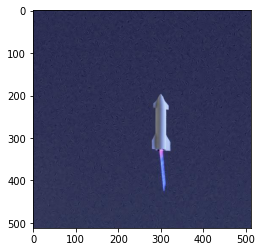In :
```gray = rgb2gray(img)
plt.imshow(gray)
```
Out:
`<matplotlib.image.AxesImage at 0x7f38053eac90>`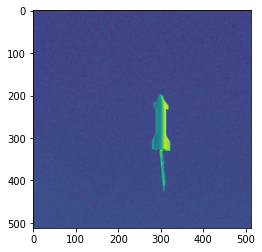In :
```result = sobel(gray)
plt.imshow(result)
```
Out:
`<matplotlib.image.AxesImage at 0x7f3804e31f50>`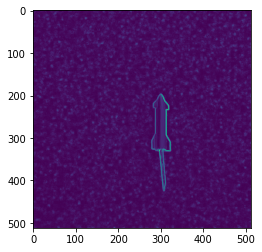In :
```contours = find_contours(result, 0.0001)

def get_bounding_rect(contour):
x_min = np.min(contour[:, 0])
x_max = np.max(contour[:, 0])
y_min = np.min(contour[:, 1])
y_max = np.max(contour[:, 1])
return round(x_min), round(y_min), round(x_max), round(y_max)

def draw_rect(img, points, color=[255, 0, 0]):
# Make sure the coordinates are expressed as integers
rr, cc = rectangle(start=points[:2], end=points[2:])
img[rr, cc] = color
return img

x_min = 512
y_min = 512
x_max = 0
y_max = 0
draw_img = img.copy()
for contour in contours:
points = get_bounding_rect(contour)
x_min = min(x_min, points)
y_min = min(y_min, points)
x_max = max(x_max, points)
y_max = max(y_max, points)
print(x_min, y_min, x_max, y_max)
draw_img = draw_rect(draw_img, [x_min, y_min, x_max, y_max])
plt.imshow(draw_img)
```
```0 0 511 511
```
Out:
`<matplotlib.image.AxesImage at 0x7f380471e5d0>`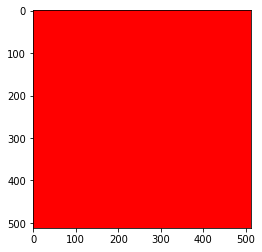### Countours¶

Contours are lines joining along the bounding of a intensity or color in an image. In the canny image or the original image, we see that the starship has much different color as compared to the sky.

So as you can see, fnding contours did a pretty great job in finfing the starship body. However there are some mistakes with the image in such as the more right side of the starship body in left unchecked.

# Submitting Results 📄¶

In :
```# Getting all the testing images
test_imgs = natsorted(os.listdir("images"))
print(len(test_imgs))
```
```10000
```
In :
```# Function to generate the bounding boxes
def gen_bounding_boxes(img):

# Converting the image to numpy array
gray = rgb2gray(img)
result = sobel(gray)

contours = find_contours(result, 0.05)

def get_bounding_rect(contour):
x_min = np.min(contour[:, 0])
x_max = np.max(contour[:, 0])
y_min = np.min(contour[:, 1])
y_max = np.max(contour[:, 1])
return round(x_min), round(y_min), round(x_max), round(y_max)

def draw_rect(img, points, color=[255, 0, 0]):
# Make sure the coordinates are expressed as integers
rr, cc = rectangle(start=points[:2], end=points[2:])
img[rr, cc] = color
return img

x_min = 512
y_min = 512
x_max = 0
y_max = 0
draw_img = img.copy()
for contour in contours:
points = get_bounding_rect(contour)
x_min = min(x_min, points)
y_min = min(y_min, points)
x_max = max(x_max, points)
y_max = max(y_max, points)

return y_min, x_min, y_max - y_min, x_max - x_min
```
In :
```bboxes = []
image_ids = []

# Ground through each test image
for img_name in tqdm(test_imgs):

# Generating the bounding boxes
x,y,w,h = gen_bounding_boxes(img)

# Adding the boundong boxes and image id
bboxes.append([x,y,w,h])
image_ids.append(int(img_name.split(".")))
```
In :
```fill = True
xs = []
ys = []
ws = []
hs = []
missing_idxes = []
for idx, bbox in enumerate(bboxes):
x, y, w, h = bbox
if x == 512 or x == 0 or y == 512 or y == 0 or w <=0 or h <= 0:
print(idx)
missing_idxes.append(idx)
else:
xs.append(x)
ys.append(y)
ws.append(w)
hs.append(h)
if fill:
x_mean = sum(xs) / len(xs)
y_mean = sum(ys) / len(ys)
w_mean = sum(ws) / len(ws)
h_mean = sum(hs) / len(hs)
for idx in missing_idxes:
bboxes[idx] = [x_mean, y_mean, w_mean, h_mean]
```
In [ ]:
```from PIL import Image, ImageDraw

def visualize(index):
image_id = image_ids[index]
bbox = bboxes[index]
x, y, w, h = bbox
draw_img = Image.open(f"images/{image_id}.jpg")
draw = ImageDraw.Draw(draw_img)
draw.rectangle([x, y, x+w, y+h], outline ="red")
return draw_img
```
In :
```visualize(182)
```
Out: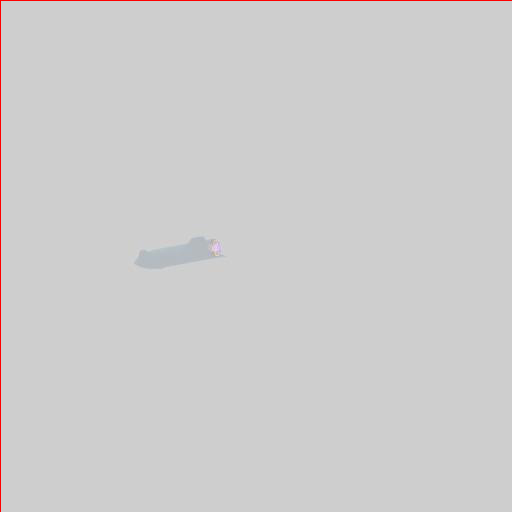In :
```visualize(2)
```
Out: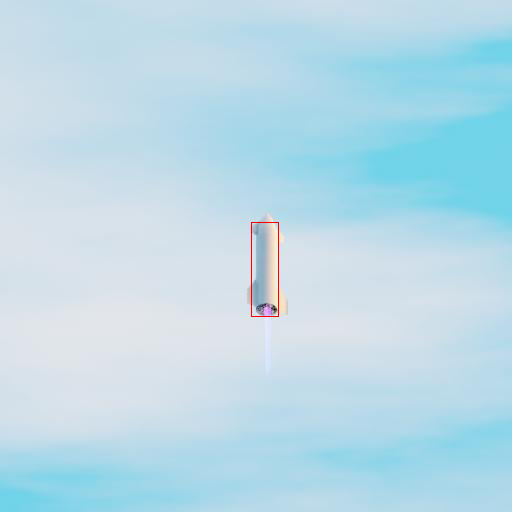In :
```visualize(100)
```
Out: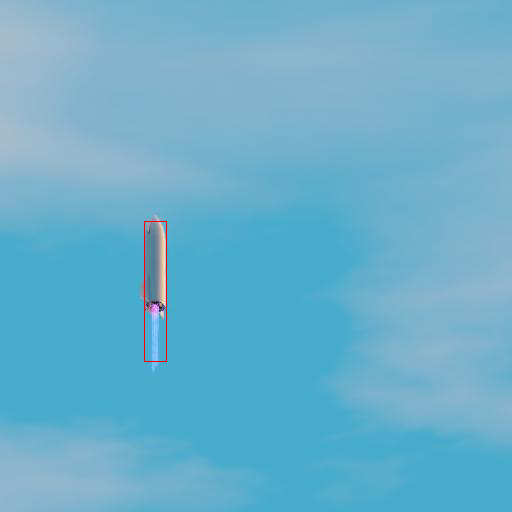In [ ]:
```def merge_boxes(boxes):
```
In :
```# Adding the image id and bounding boxes to a dataframe
df = pd.DataFrame({"ImageID":image_ids, "bbox":bboxes})
df = df.sort_values("ImageID").reset_index(drop=True)
df
```
Out:
ImageID bbox
0 0 [167, 215, 20, 98]
1 1 [273, 227, 128, 41]
2 2 [251, 222, 27, 94]
3 3 [203, 242, 169, 34]
4 4 [321, 214, 34, 104]
... ... ...
9995 9995 [204, 239, 183, 28]
9996 9996 [182, 244, 67, 21]
9997 9997 [95, 207, 203, 81]
9998 9998 [159, 237, 22, 80]
9999 9999 [164, 215, 25, 113]

10000 rows × 2 columns

In :
```!rm -rf assets
!mkdir assets

df.to_csv(os.path.join("assets", "submission.csv"), index=False)
```

Note : Please make sure that there should be filename `submission.csv` in `assets` folder before submitting it

In :
```
```
```Using notebook: /content/drive/MyDrive/Colab Notebooks/Starship Detection SKImage for submission...
Removing existing files from submission directory...
Scrubbing API keys from the notebook...
Collecting notebook...
submission.zip ━━━━━━━━━━━━━━━━━━━━ 100.0% • 785.2/783.5 KB • 1.3 MB/s • 0:00:00
╭─────────────────────────╮
│ Successfully submitted! │
╰─────────────────────────╯
┌──────────────────┬───────────────────────────────────────────────────────────────────────────────────────────────────────────┐
│  This submission │ https://www.aicrowd.com/challenges/ai-blitz-x/problems/starship-detection/submissions/152143              │
│                  │                                                                                                           │
│  All submissions │ https://www.aicrowd.com/challenges/ai-blitz-x/problems/starship-detection/submissions?my_submissions=true │
│                  │                                                                                                           │
│                  │                                                                                                           │
│ Discussion forum │ https://discourse.aicrowd.com/c/ai-blitz-x                                                                │
│                  │                                                                                                           │
│   Challenge page │ https://www.aicrowd.com/challenges/ai-blitz-x/problems/starship-detection                                 │
└──────────────────┴───────────────────────────────────────────────────────────────────────────────────────────────────────────┘
```
In [ ]:
```
```
163